Updating search results...

# 40 Results

View
Selected filters:
• Virtual Laboratories in Probability and Statistics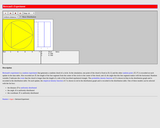Unrestricted Use
CC BY
Rating
0.0 stars

This resource consists of a Java applet and expository text. The applet is a simulation of Bertrand's experiment: a random chord on a circle The event of interest is whether the length of the chord is larger than the length of the inscribed equilateral triangle. Three models for generating the random chord can be used.

Subject:
Mathematics
Statistics and Probability
Material Type:
Activity/Lab
Interactive
Simulation
Provider:
University of Alabama in Huntsville
Provider Set:
Virtual Laboratories in Probability and Statistics
Author:
Kyle Siegrist
02/16/2011Unrestricted Use
CC BY
Rating
0.0 stars

This resource consists of a Java applet and expository text. The applet illustrates Bayesian estimation of the probability of heads for a coin. The prior beta distribution, true probability of heads, and the sample size can be specified. The applet shows the posterior beta distribution.

Subject:
Mathematics
Statistics and Probability
Material Type:
Activity/Lab
Interactive
Simulation
Provider:
University of Alabama in Huntsville
Provider Set:
Virtual Laboratories in Probability and Statistics
Author:
Kyle Siegrist
02/16/2011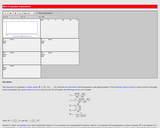Unrestricted Use
CC BY
Rating
0.0 stars

This resource consists of a Java applet and expository text. The applet simulates a random sample from a beta distribution, and computes standard point estimates of the left and right parameters. The bias and mean square error are also computed.

Subject:
Mathematics
Statistics and Probability
Material Type:
Activity/Lab
Interactive
Simulation
Provider:
University of Alabama in Huntsville
Provider Set:
Virtual Laboratories in Probability and Statistics
Author:
Kyle Siegrist
02/16/2011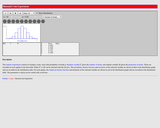Unrestricted Use
CC BY
Rating
0.0 stars

This resource consist of a Java applet and expository text. The applet simulates Bernoulli trials in terms of coin tosses. The random variables of interest are the number of heads and the proportion of heads. The number of coins and the probability of heads can be varied. The applet illustrates the law of large numbers and the central limit theorem.

Subject:
Mathematics
Statistics and Probability
Material Type:
Activity/Lab
Interactive
Simulation
Provider:
University of Alabama in Huntsville
Provider Set:
Virtual Laboratories in Probability and Statistics
Author:
Kyle Siegrist
02/16/2011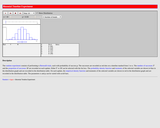Unrestricted Use
CC BY
Rating
0.0 stars

This resource consists of a Java applet and expository text. The applet simulates Bernoulli trials in terms of random points on a timeline. The random variables of interest are the number of successes and the proportion of successes. The number of trials and the probability of success can be varied. This applet illustrates the law of large numbers, the central limit theorem, and the binomial distribution.

Subject:
Mathematics
Statistics and Probability
Material Type:
Activity/Lab
Interactive
Simulation
Provider:
University of Alabama in Huntsville
Provider Set:
Virtual Laboratories in Probability and Statistics
Author:
Kyle Siegrist
02/16/2011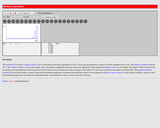Unrestricted Use
CC BY
Rating
0.0 stars

This resource consists of a Java applet and expository text. The applet is a simulation of the birthday experiment: a sample of size n is chose at random and with replacement from the first m positive integers. The random variable of interest is the number of distinct sample values. The event of interest is that all sample values are distinct.

Subject:
Mathematics
Statistics and Probability
Material Type:
Activity/Lab
Interactive
Simulation
Provider:
University of Alabama in Huntsville
Provider Set:
Virtual Laboratories in Probability and Statistics
Author:
Kyle Siegrist
02/16/2011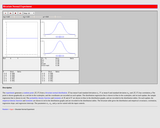Unrestricted Use
CC BY
Rating
0.0 stars

This resource consists of a Java applet and expository text. The applet simulates the bivariate normal distribution. The means are set at 0, but the standard deviations and the correlation can be varied. Simulated points from the distribution are shown as dots in a scatterplot.

Subject:
Mathematics
Statistics and Probability
Material Type:
Activity/Lab
Interactive
Simulation
Provider:
University of Alabama in Huntsville
Provider Set:
Virtual Laboratories in Probability and Statistics
Author:
Kyle Siegrist
02/16/2011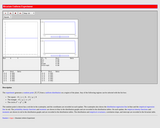Unrestricted Use
CC BY
Rating
0.0 stars

This resource consists of a Java applet and expository text. The Java applet illustrates the bivariate uniform distribution on three types of regions: a square, a circle, and a triangle. Simulated points from the distribution are shown as dots in a scatterplot.

Subject:
Mathematics
Statistics and Probability
Material Type:
Activity/Lab
Interactive
Simulation
Provider:
University of Alabama in Huntsville
Provider Set:
Virtual Laboratories in Probability and Statistics
Author:
Kyle Siegrist
02/16/2011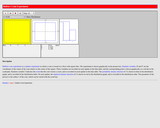Unrestricted Use
CC BY
Rating
0.0 stars

This resource consists of a Java applet and expository text. The applet simulates Buffon's coin experiment. The radius of the coin can be varied. The applet illustrates a random experiment, the sample space, random variables, events, probability, and relative frequency.

Subject:
Mathematics
Statistics and Probability
Material Type:
Activity/Lab
Interactive
Simulation
Provider:
University of Alabama in Huntsville
Provider Set:
Virtual Laboratories in Probability and Statistics
Author:
Kyle Siegrist
02/16/2011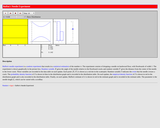Unrestricted Use
CC BY
Rating
0.0 stars

This resource consists of a Java applet and expository text. The applet simulates Buffon's needle experiment and the corresponding approximation of pi. The event of interest is that the needle crosses a crack. The length of the needle can be varied. The applet illustrates a random experiment, the sample space, random variables, probability, and relative frequency.

Subject:
Mathematics
Statistics and Probability
Material Type:
Activity/Lab
Interactive
Simulation
Provider:
University of Alabama in Huntsville
Provider Set:
Virtual Laboratories in Probability and Statistics
Author:
Kyle Siegrist
02/16/2011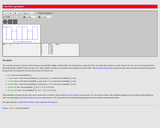Unrestricted Use
CC BY
Rating
0.0 stars

This resource consists of a Java applet and expository text. The applet is a simulation of the experiment that consists of tossing a coin and then rolling either a red die or a green die, depending on the outcome of the coin toss. The probability of heads and the distributions of the two dice can be specified. The applet illustrates a two-stage experiment.

Subject:
Mathematics
Statistics and Probability
Material Type:
Activity/Lab
Interactive
Simulation
Provider:
University of Alabama in Huntsville
Provider Set:
Virtual Laboratories in Probability and Statistics
Author:
Kyle Siegrist
02/16/2011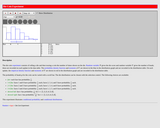Unrestricted Use
CC BY
Rating
0.0 stars

This resource consists of a Java applet and expository text. The applet simulates the experiment of rolling a die and then tossing a coin the number of times shown on the die. The die distribution and the probability of heads can be specified. The applet illustrates a two-stage experiment.

Subject:
Mathematics
Statistics and Probability
Material Type:
Activity/Lab
Interactive
Simulation
Provider:
University of Alabama in Huntsville
Provider Set:
Virtual Laboratories in Probability and Statistics
Author:
Kyle Siegrist
02/16/2011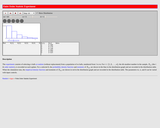Unrestricted Use
CC BY
Rating
0.0 stars

This resource consists of a Java applet and expository text. The applet is a simulation of the experiment of selecting n objects at random from the first m positive integers. The random variables of interest are the order statistics. The applet illustrates the distributions of the order statistics.

Subject:
Mathematics
Statistics and Probability
Material Type:
Activity/Lab
Interactive
Simulation
Provider:
University of Alabama in Huntsville
Provider Set:
Virtual Laboratories in Probability and Statistics
Author:
Kyle Siegrist
02/16/2011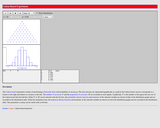Unrestricted Use
CC BY
Rating
0.0 stars

This resource consists of a Java applet and descriptive text. The applet illustrates Bernoulli trials in terms of a Galton Board. The number of trials and the probability of success can be varied. The random variables of interest are the number of successes and the probability of a success. The applet illustrates the binomial distribution, the law of large numbers and the central limit theorem.

Subject:
Mathematics
Statistics and Probability
Material Type:
Activity/Lab
Interactive
Simulation
Provider:
University of Alabama in Huntsville
Provider Set:
Virtual Laboratories in Probability and Statistics
Author:
Kyle Siegrist
02/16/2011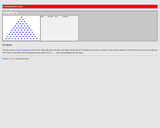Unrestricted Use
CC BY
Rating
0.0 stars

This resource consists of a Java applet and expository text. The applet illustrates the Galton board in which a ball falls through a triangular array of pegs. Various combinatorial ideas are illustrated, including combinations, bit strings, and binomial coefficients.

Subject:
Mathematics
Statistics and Probability
Material Type:
Activity/Lab
Interactive
Simulation
Provider:
University of Alabama in Huntsville
Provider Set:
Virtual Laboratories in Probability and Statistics
Author:
Kyle Siegrist
02/16/2011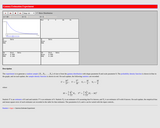Unrestricted Use
CC BY
Rating
0.0 stars

This resource consists of a Java applet and expository text. The applet simulates a random sample from a gamma distribution, and computes standard point estimates of the shape and scale parameters. The bias and mean square error are also computed.

Subject:
Mathematics
Statistics and Probability
Material Type:
Activity/Lab
Interactive
Simulation
Provider:
University of Alabama in Huntsville
Provider Set:
Virtual Laboratories in Probability and Statistics
Author:
Kyle Siegrist
02/16/2011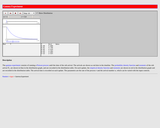Unrestricted Use
CC BY
Rating
0.0 stars

This resource consists of a Java applet and expository text. The applet simulates the time of the k'th arrival in a Poisson process. The arrival number k and the rate of the Poisson process can be varied. The applet illustrates the gamma distribution and a special case of the central limit theorem.

Subject:
Mathematics
Statistics and Probability
Material Type:
Activity/Lab
Simulation
Provider:
University of Alabama in Huntsville
Provider Set:
Virtual Laboratories in Probability and Statistics
Author:
Kyle Siegrist
02/01/2006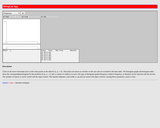Unrestricted Use
CC BY
Rating
0.0 stars

This resource consists of a Java applet and expository text. The applet allows the user to construct a histogram by clicking on a number line to generate the sample data. The class width can be varied and various summary statistics can be displayed.

Subject:
Mathematics
Statistics and Probability
Material Type:
Activity/Lab
Simulation
Provider:
University of Alabama in Huntsville
Provider Set:
Virtual Laboratories in Probability and Statistics
Author:
Kyle Siegrist
02/20/2006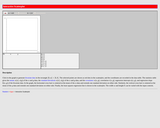Unrestricted Use
CC BY
Rating
0.0 stars

This resource consists of a Java applet and expository text. The applet allows the user to construct a scatterplot by clicking on a graph to generate the bivariate data. The means, standard deviations, correlation, and the regression line are shown.

Subject:
Mathematics
Statistics and Probability
Material Type:
Activity/Lab
Interactive
Simulation
Provider:
University of Alabama in Huntsville
Provider Set:
Virtual Laboratories in Probability and Statistics
Author:
Kyle Siegrist
02/16/2011Unrestricted Use
CC BY
Rating
0.0 stars

This resource consists of a Java applet and expository text. The applet is a simulation of the matching experiment, which consists of a random permutation of the first n positive integers. The random variable of interest is the number of matches (the number of integers in the random permutation that are in their correct positions). The applet illustrates the distribution of the number of matches and the convergence to the Poisson distribution.

Subject:
Mathematics
Statistics and Probability
Material Type:
Activity/Lab
Interactive
Simulation
Provider:
University of Alabama in Huntsville
Provider Set:
Virtual Laboratories in Probability and Statistics
Author:
Kyle Siegrist